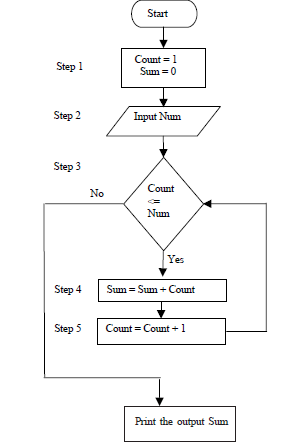#### Flow Charting of a Algorithm

Flow Charting

A flow chart is pictorial representation of an algorithm. It gives an easy and clear understanding of an algorithm. The understanding of an algorithm is made easy by flow charts, as compared to textual representation of an algorithm.

Flow Chart Symbols

Flow chart has many symbols for basic operation in programming. The flow chart symbols are:

i) Terminal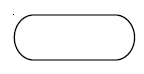This is usually to represent terminal points in a program like BEGIN, END, START, and STOP.

ii) Input/output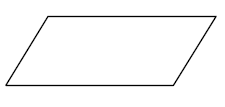The parallelogram represents the Input/output function i.e. making the data available for processing (input) or recording of the processed information (output). This step implies obtaining a number from an input device (say, the keyboard and storing it in the storage location named 'A')

iii) Process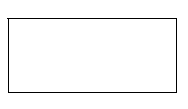The rectangle represents the processing operation. A process changes the data. An assignment is usually represented by this symbol.

iv) Flow direction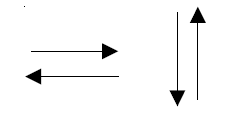Lines or arrows represent the flow direction - the flow of control. Normally the flow direction is from left to right or top to bottom.

v) Annotation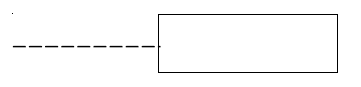A broken line and rectangle represent the annotation function - the addition of descriptive comments or explanatory notes for clarification of some statements.

vi) Decision making symbol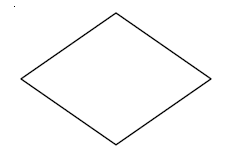The diamond represents a decision or switching type of operation that determines which of the alternative paths is to be followed. A decision points out a question that can be answered yes or no (or true or false).

vii) Connector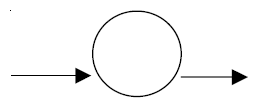A Circle is connector symbol which is used to represent functions in a flow line i.e. the part of flow chart which continues in the next or another page.

viii) Pre-defined process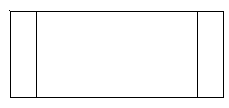The double sided rectangle presents a named procedure that consists of one or more operations or programming steps that are precised elsewhere such as a module or subroutine.

Flow Charting of an Algorithm

A flow chart can be implemented after the algorithm is defined just by following the steps and making appropriate symbols and texts in them. It is illustrated with an example here:

Flow chart for the example of adding n numbers is given below:

Step 1 Initialize variables Count to 1 and Sum to 0

Step 2 Input the number till what value the sum has to be performed as Num

Step 3 Repeat the steps 4 and 5 till value of Count is less than or equal to Num

Step 4 Add Sum and Count and assign the result to Sum

Step 5 Increment Count by 1.